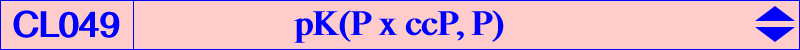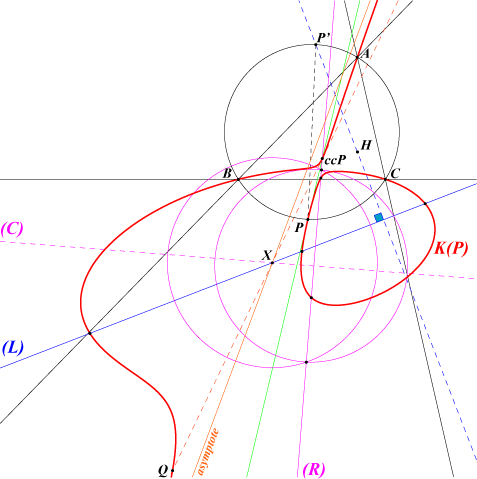Let P = u:v:w be a finite point distinct of G and ccP = v + w + 2u : : , the complement of the complement of P.   Any pK with pivot P, isopivot ccP, pole P x ccP = u(v + w + 2u) : : , is a pK+ with three (real or not) asymptotes concurring at X = u(v + w)(v + w + 2u) : : , the barycentric product of ccP and ctP. ctP is the complement of the isotomic conjugate of P i.e. the center of the inconic with perspector P. X is also the homothetic under h(ccP, 1/3) of Q = P / ccP (cevian quotient), the tangential of ccP in the cubic.   The most interesting cubic of the family is probably the McCay orthic cubic K049 = pK(X53, X4) since it is the only pK60+ of this class of cubics. See other pK60+ in CL006. pK(P x ccP, P) with P on the circumcircle This class CL049 contains a remarkable subclass of cubics obtained when P lies on the circumcircle. Indeed, each cubic K(P) of this type has a pencil of circular polar conics. In other words, there is a line (L) – we call the circular line of the cubic – such that the polar conic of each point M on (L) is a circle. Obviously, these circles belong to a same pencil. (L) contains X and is perpendicular to the line passing through H and the antipode P' of P on the circumcircle. When P traverses the circumcircle, (L) envelopes a conic homothetic to the McBeath conic (the inconic with foci O and H) with center on the Euler line and on the line X(206)X(524). The polar conic of X decomposes into the line at infinity and the radical axis (R) of the circles which is the line passing through the pivot ccP and the pole P x ccP of K(P). These circles are therefore centered on the perpendicular (C) at X to the radical axis.K602 and K603 are two examples of such cubics. See CL009 for other pKs with circular polar conics and also Table 47.